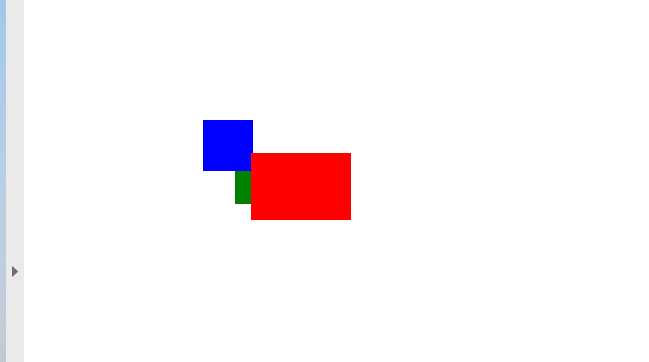# Add Rectangle Object to PDF file

Contents
[ ]

Aspose.PDF for .NET supports the feature to add graph objects (for example graph, line, rectangle etc.) to PDF documents. You also get the leverage to add Rectangle object where you also offers the feature to fill rectangle object with a certain color, control Z-Order, add gradiant color fill and etc.

First, let’s look at the possibility of creating a Rectangle object.

1. Create a new PDF Document

2. Add Page to pages collection of PDF file

3. Add Text fragment to paragraphs collection of page instance

4. Create Graph instance

5. Set border for Drawing object

6. Create Rectangle instance

7. Add Rectangle object to shapes collection of Graph object

8. Add graph object to paragraphs collection of page instance

9. Add Text fragment to paragraphs collection of page instance

10. And save your PDF file

`````` private static void AddRectangle(Page page, float x, float y, float width, float height, Color color, int zindex)
{
// Create graph object with dimensions same as specified for Rectangle object
Aspose.Pdf.Drawing.Graph graph = new Aspose.Pdf.Drawing.Graph(width, height)
{
// Can we change the position of graph instance
IsChangePosition = false,
// Set Left coordinate position for Graph instance
Left = x,
// Set Top coordinate position for Graph object
Top = y
};
// Add a rectangle inside the "graph"
Rectangle rect = new Rectangle(0, 0, width, height);
// Set rectangle fill color
rect.GraphInfo.FillColor = color;
// Color of graph object
rect.GraphInfo.Color = color;
// Add rectangle to shapes collection of graph instance
// Set Z-Index for rectangle object
graph.ZIndex = zindex;
// Add graph to paragraphs collection of page object
}
``````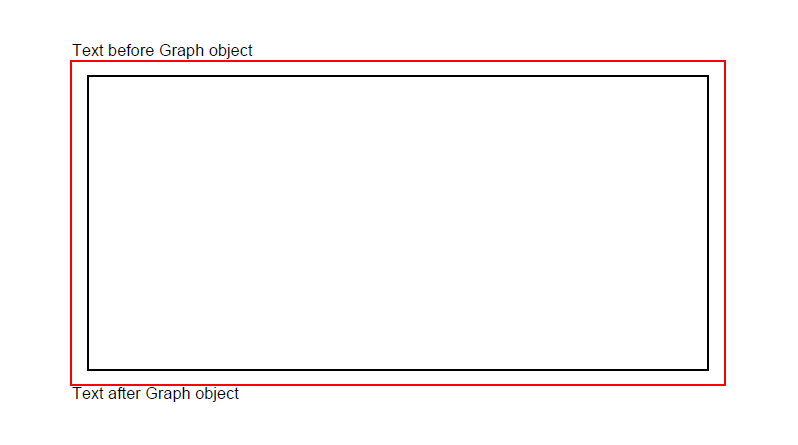## Create Filled Rectangle Object

Aspose.PDF for .NET also offers the feature to fill rectangle object with a certain color.

The following code snippet shows how to add a Rectangle object that is filled with color.

``````    {
private const string _dataDir = "C:\\Samples\\";
public static void RectangleFilled()
{
// Create Document instance
var doc = new Document();

// Add page to pages collection of PDF file
// Create Graph instance
var graph = new Aspose.Pdf.Drawing.Graph(100, 400);

// Add graph object to paragraphs collection of page instance

// Create Rectangle instance
var rect = new Rectangle(100, 100, 200, 120);

// Specify fill color for Graph object
rect.GraphInfo.FillColor = Color.Red;

// Add rectangle object to shapes collection of Graph object

// Save PDF file
}
``````

Look at the result of rectangle filled solid color: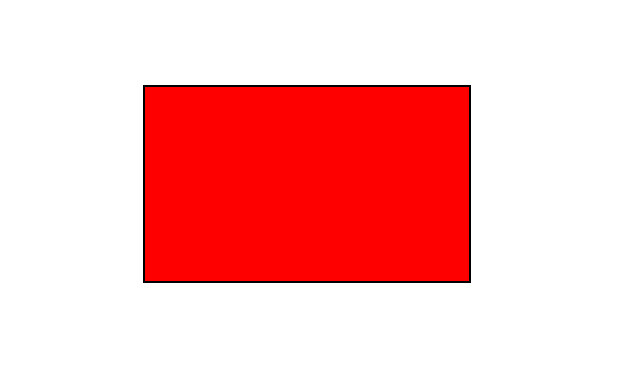Aspose.PDF for .NET supports the feature to add graph objects to PDF documents and sometimes it is required to fill graph objects with Gradient Color. To Fill graph objects with Gradient Color, We need to set setPatterColorSpace with gradientAxialShading object as following.

The following code snippet shows how to add a Rectangle object that is filled with Gradient Color.

`````` public static void CreateFilledRectangletGradientFill()
{
// Create Document instance
var doc = new Document();

// Add page to pages collection of PDF file
// Create Graph instance
var graph = new Aspose.Pdf.Drawing.Graph(400, 400);
// Add graph object to paragraphs collection of page instance
// Create Rectangle instance
var rect = new Rectangle(0, 0, 300, 300);
// Specify fill color for Graph object
{
Start = new Point(0, 0),
End = new Point(350, 350)
};

// Add rectangle object to shapes collection of Graph object

// Save PDF file
}
``````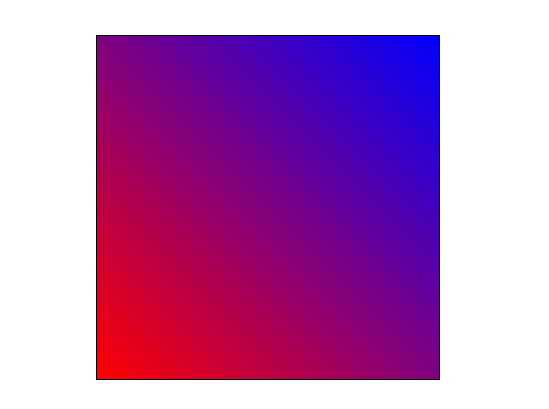## Create Rectangle with Alpha color channel

Aspose.PDF for .NET supports to fill rectangle object with a certain color. A rectangle object can also have Alpha color channel to give transparent appearance. The following code snippet shows how to add a Rectangle object with Alpha color channel.

Pixels of the image can store information about their opacity along with color value. This allows creating images with transparent or semi-transparent areas.

Instead of making a color transparent, each pixel stores information on how opaque it is. This opacity data is called alpha channel and is typically stored after the color channels of the pixel.

``````     public static void RectangleFilled_AlphaChannel()
{
// Create Document instance
var doc = new Document();

// Add page to pages collection of PDF file
// Create Graph instance
var graph = new Aspose.Pdf.Drawing.Graph(100, 400);
// Add graph object to paragraphs collection of page instance
// Create Rectangle instance
var rect = new Rectangle(100, 100, 200, 120);
// Specify fill color for Graph object
rect.GraphInfo.FillColor = Color.FromArgb(128, 244, 180, 0);

// Add rectangle object to shapes collection of Graph object

// Create second rectangle object
var rect1 = new Rectangle(200, 150, 200, 100);
rect1.GraphInfo.FillColor = Color.FromArgb(160, 120, 0, 120);

// Add graph instance to paragraph collection of page object

// Save PDF file
}
``````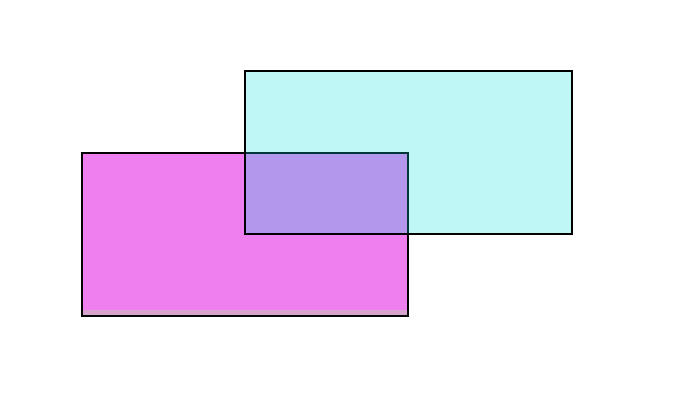## Control Z-Order of Rectangle

Aspose.PDF for .NET supports the feature to add graph objects (for example graph, line, rectangle etc.) to PDF documents. When adding more than one instance of same object inside PDF file, we can control their rendering by specifying the Z-Order. Z-Order is also used when we need to render objects on top of each other.

The following code snippet shows the steps to render Rectangle objects on top of each other.

`````` public static void AddRectangleZOrder()
{
// Instantiate Document class object
Document doc1 = new Document();
/// Add page to pages collection of PDF file
// Set size of PDF page
page1.SetPageSize(375, 300);
// Set left margin for page object as 0
page1.PageInfo.Margin.Left = 0;
// Set top margin of page object as 0
page1.PageInfo.Margin.Top = 0;
// Create a new rectangle with Color as Red, Z-Order as 0 and certain dimensions
AddRectangle(page1, 50, 40, 60, 40, Color.Red, 2);
// Create a new rectangle with Color as Blue, Z-Order as 0 and certain dimensions
AddRectangle(page1, 20, 20, 30, 30, Color.Blue, 1);
// Create a new rectangle with Color as Green, Z-Order as 0 and certain dimensions
AddRectangle(page1, 40, 40, 60, 30, Color.Green, 0);
// Save resultant PDF file